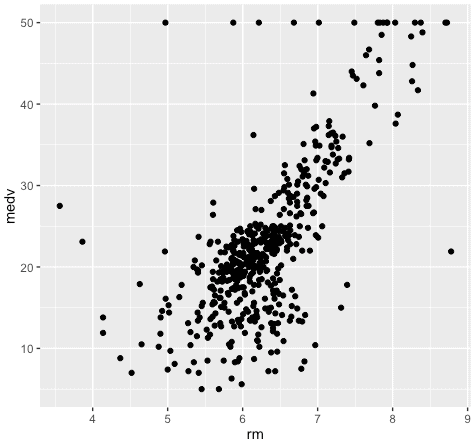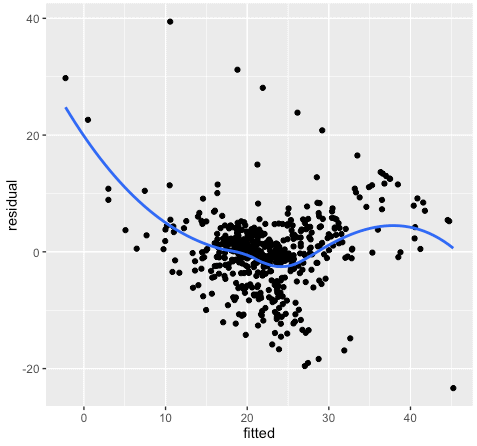Want to share your content on R-bloggers? click here if you have a blog, or here if you don't.

In part 1, we went over how to use data visualization and data analysis prior to machine learning. For example, we discussed how to visualize the data to identify potential issues in the dataset, examine the variable distributions, etc.

In this blog post, we’ll continue by building a very simple model and using data visualization to examine that model.

Just a quick reminder: as I noted in part 1, we’re working with a very simple model. This is deliberately a “toy” model, which allows us to focus on the visualization/analysis aspect of the task without the added level of complexity that we’d inject by using a more advanced machine learning algorithm.

Having said that, let’s dive in.

## Build a simple linear regression model

Having explored our data in part 1, let’s continue our work by building a basic linear regression model.

We’ll build a simple linear regression model by regressing medv onto rm. Effectively, we’re going to fit a straight line on top of the following data:Let’s create the linear regression model using the lm() function:

#-----------
# GET DATA
#-----------

data(Boston, package = "MASS")

#------------------------
# BUILD MODEL USING lm()
#------------------------

lm.boston_simple <- lm(medv ~ rm
,data = Boston
)


## Plot the model

Now that we’ve built the model, let’s quickly plot it.

To do this, we’re going to:

1. Extract the coefficients from our model object using the coef()function. Note here that I’m using the prefix “coef.“. This prefix is meaningless syntactically, but I use prefixes like these to help keep my objects organized. It’s slightly unconventional, but it absolutely helps with ‘bookkeeping’ and keeping track of objects.

2. Plot the data and model using ggplot
Here, we’ll just plot the data points using geom_point(), and then add the regression line as an additional layer using geom_abline().

Keep in mind that in this case, we can plot the model because we have only two variables (one predictor and one target). That is, because we only have two variables to visualize, we can visualize it with a scatterplot. However, in many cases, you’ll build models with many predictors and there won’t be a way to directly visualize the model in many dimensions.

#-------------------------------
# PLOT LINEAR MODEL ON SCATTER
#-------------------------------

# EXTRACT MODEL COEFFICIENTS

coef.lm_icept <- coef(lm.boston_simple)
coef.lm_slope <- coef(lm.boston_simple)

# PLOT MODEL AND POINTS

require(ggplot2)
ggplot() +
geom_point(data = Boston, aes(x = rm, y = medv)) +
geom_abline(intercept = coef.lm_icept, slope = coef.lm_slope, color = "red")Visually, this model looks like an OK fit. We can see that the regression line cuts through the bulk of the points, but there are several points that are far away from the regression line. That is, there are quite a few large residuals.

## Residual plots and diagnostics

Now that we’ve looked at a plot of the model against the data, let’s use some additional visualization techniques to evaluate the model. To do this, we’ll use residual plots.

Residual plots can be very useful for evaluating how well the model fits the data, as noted by Kuhn and Johnson in their excellent book, Applied Predictive Modeling:

Visualizations of the model fit, particularly residual plots, are critical to understanding whether the model is fit for purpose.”

One thing that we can evaluate with residual plots is whether or not there is a linear relationship between the predictor(s) and the response. For linear regression, we’re making a strong assumption about the relationship between the target and the predictors. We’re assuming that there’s a linear relationship. In the case of simple linear regression, we’re assuming a relationship of the form(although in the case of multiple regression, we assume a hyperplane instead of a line).

This is a strong assumption that doesn’t always hold.

How do we know? How do we verify that the relationship is linear?

### Plot: fitted values vs. residuals

Residual plots are an excellent diagnostic tool for validating this assumption. A plot of the predicted value vs the residual can help us identify a possible non-linear relationship. If we find a non-linear relationship, this is an indication that the linear model is not a good fit.

#------------------------------
# SCATTERPLOT: Fitted vs Resid
#------------------------------

df.lm.boston_simple <- fortify(lm.boston_simple)

# WITH LOESS SMOOTHE
ggplot(data = df.lm.boston_simple, aes(x = .fitted, y = .resid)) +
geom_point() +
stat_smooth(se = FALSE) +
labs(x = "fitted", y = "residual")If the relationship between the predictor and the response is actually linear, then the plot of fitted values vs residuals should look random, without any detectable pattern.

The data in this chart do not look random. There’s a noticeable, non-linear pattern in the plot. This is a bit of a cause for concern and an indication that we may want to modify our model with additional predictors, transformations of our predictors, etc.

### Plot: histogram of the residuals

We can also look at the histogram of the residuals. When we use a linear regression model, we want the residuals to be normally distributed, so we can visually inspect the histogram to visually identify normality or non-normality.

#-----------------------------
# DIAGNOSTIC PLOT:
#  Histogram of the Residuals
#-----------------------------

ggplot(data = df.lm.boston_simple, aes(x = .resid)) +
geom_histogram()In this plot, we can see more clearly that the residuals are not normally distributed. Again, this is an indication that the model is not a great fit, and we may want to make some modifications.

## Other uses for visualization in machine learning

As I mentioned in the beginning of the post, this is really a very simple example. Not only is simple linear regression perhaps the simplest example of machine learning, but in the interest of brevity, I’ve left out some other parts of the workflow where you might need visualization.

Having said that, I’ll give you a quick list of some additional ways that you might need visualization and analysis in ML, either for linear regression, or for more advanced techniques:

1. Model tuning
For some model types other than linear regression, you need to “tune” your model by selecting the best values for model parameters. When you perform model tuning, there are several visualization techniques that can be helpful for selecting the ideal parameters. Heat maps can be particularly useful for this purpose.

2. Learning curves
To diagnose potential problems in your model and optimize its performance, you can plot learning curves.

Even after you build your final model, if you’re in industry, you’ll have to communicate your results.

In many cases, your audience may have limited mathematical sophistication. Because of this, data visualization is frequently the best way to communicate the results of your model and explain how it works to your business patterns and management.

This is often overlooked in textbooks and tutorials, but in industry and business, it’s a huge part of your job.

## If you want to learn machine learning, learn data analysis first

Between this post and the last blog post, you should have a basic idea of how you’ll use data visualization and analysis in the machine learning workflow.

As I’ve emphasized several times, data analysis and data visualization form the foundation of more advanced data science topics. If you want to learn applied machine learning, you need to have a strong foundation in analysis and visualization.

If you’re ready to get really serious about mastering data analysis and visualization in R, I’m going to be launching a new training program very soon. It’ll enable you to rapidly master analysis and visualization. You’ll learn everything you need to know, from the ground up.

If you’re interested in the training program, sign up for our email list so you know when the program launches.

The post How to use data analysis for machine learning, part 2 appeared first on SHARP SIGHT LABS.